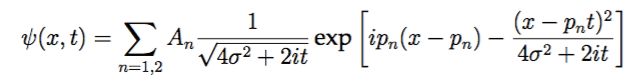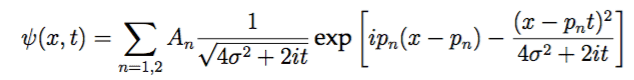# QM: Probability

• I
WWCY

## Homework Statement

I am supposed to find probability of staying in x < 0 for a superposition of two Gaussians. The wavefunction is something along the lines of:## The Attempt at a Solution

Usually, the step involved in finding probabilities for 1 particle is just to perform the integral of ##|\Psi|^2## between 2 points (##-\infty## to 0 i believe) . However, I believe this wavefunction is the sum of 2 separately normalized gaussians. I'm not sure how I should proceed.

Advice is greatly appreciated!

## Answers and Replies

Mentor
You can still calculate this integral. Just calculate the integral of both separately and add them.

WWCY
You can still calculate this integral. Just calculate the integral of both separately and add them.

Thanks for the response!

But if I choose to do both integrals from -infinity to infinity, and sum them, does that not give me a probability of 2?

Homework Helper
Dearly Missed

## Homework Statement

I am supposed to find probability of staying in x < 0 for a superposition of two Gaussians. The wavefunction is something along the lines of:

View attachment 214386

## The Attempt at a Solution

Usually, the step involved in finding probabilities for 1 particle is just to perform the integral of ##|\Psi|^2## between 2 points (##-\infty## to 0 i believe) . However, I believe this wavefunction is the sum of 2 separately normalized gaussians. I'm not sure how I should proceed.

Advice is greatly appreciated!

The probability that ##x < 0## at time ##t## is ##\Pr(x < 0|t) = P(t) = \int_{-\infty}^0 |\psi(x,t)|^2 \, dx##, so you need to write out the four terms of ##|\psi|^2 = \psi^* \, \psi## and then integrate them separately. It will be complicated and unpleasant.

However, I am not quite sure what the statement "staying in ##x < 0##" means. Interpreted literally, it is asking for the probability that ##x<0## for all ##t##, and this is very different from asking that ##x < 0## for any single value of ##t##. Is that really what is wanted?

WWCY
The probability that ##x < 0## at time ##t## is ##\Pr(x < 0|t) = P(t) = \int_{-\infty}^0 |\psi(x,t)|^2 \, dx##, so you need to write out the four terms of ##|\psi|^2 = \psi^* \, \psi## and then integrate them separately. It will be complicated and unpleasant.

Will this not give me something unphysical? In the problem, these Gaussians were normalized separately, with A being their normalization constants, if I chose to do the integral from ##-\infty## to ##\infty##, would it not give me something > 1?

However, I am not quite sure what the statement "staying in ##x < 0##" means. Interpreted literally, it is asking for the probability that ##x<0## for all ##t##, and this is very different from asking that ##x < 0## for any single value of ##t##. Is that really what is wanted?

Yep, I was asked to investigate how the probability for the region ##-\infty## to 0 evolved with time, so I do need an expression for all ##t##. To provide some context, all of this is part of a project about quantum backflow, and I'm tasked to do the "simpler" calculations like the ones above.

Thanks for the assistance!

Homework Helper
Gold Member
staying in x<0" means. Interpreted literally, it is asking for the probability that x<0 for all t
Quite. I wonder if it was intended to ask for the probability at t=∞.
these Gaussians were normalized separately
Then you do not have enough information. You need to know the proportions in which to combine them before renormalising.

Mentor
If the given wavefunction is properly normalized, then the normalization constants take care that the total integral over the squared wave function is 1. This means ##|A_1|^2 + |A_2|^2 = 1##. An integral over a smaller x range will then give a value smaller than 1.

If the question asks for ##t \to \infty##, you'll need a different approach. Hint: Look at the momentum distribution.

WWCY
Hi all, thanks for the responses, I think it's best for me to upload the paper I'm referring to.

https://arxiv.org/pdf/1301.4893.pdf

All of the stuff that I'm referring to is on pages 4 and 5 of the document. In particular, I'm looking to recover analytic expressions for Figures 1. (Probability Current) and 2. (Probability from ##-\infty<x<0##).

For probability current, I'm assuming that it's just a case of plugging the wavefunction into the Current equation.

I'd really appreciate it if someone could look through it and advise me on the interpretation!

•mfb
WWCY
Then you do not have enough information. You need to know the proportions in which to combine them before renormalising.

Do you mean something like ##\frac{1}{\sqrt{2}} \psi_1 + \frac{1}{\sqrt{2}} \psi_2##?

WWCY
I initially made a post in the HW section about this topic but I believe that my question was poorly formulated, hence I'm attempting to ask a better one here, since it isn't exactly HW.

I am studying the probability current, and probability of a sum of 2 gaussians. The type of wavefunction is as shown :where ##\hbar = m = 1##

The ultimate goals are to:
a) Obtain an analytic expression for Probability Current at x = 0
b) Obtain an analytic expression for probability from ##-\infty < x < 0## for all ##t## - to study how the probability of the two wavepackets remaining at ##x<0## changes with time.
c) To obtain plots similar to Figures 1 (Probability Current) and 2 (Probability) in this paper (pages 4 and 5 respectively): https://arxiv.org/pdf/1301.4893.pdf

What I intend to do:

I was given an initial Gaussian of: ##\Psi(x,0) = e^{ipx}e^{\frac{-x^2}{2\sigma ^2}}##.

Normalizing, finding ##\widetilde{\psi}## and then ##\Psi(x,t)## for 1 Gaussian gives,
$$\Psi_k = \frac{\sqrt{\sigma}}{\pi^{1/4} \sqrt{\sigma ^2 + it}}exp(ip_k (x - \frac{p_k}{2}t) + \frac{(x - p_k t)^2}{2(\sigma ^2 + it)})$$
then summing over 2 Gaussians will give,
$$\Psi_k =\sum_{k=1,2} \frac{\sqrt{\sigma}}{\pi^{1/4} \sqrt{\sigma ^2 + it}}exp(ip_k (x - \frac{p_k}{2}t) + \frac{(x - p_k t)^2}{2(\sigma ^2 + it)})$$
which is not dissimilar to the one above, except for the fact that I should have started with ##\Psi = e^{ipx}e^{\frac{-x^2}{4\sigma ^2}}##, I believe.

For a: I intend to place the Sum of Gaussians into the Probability Current equation, and chug through the algebra to derive the expression, evaluating the expression at x = 0.

For b: This is the part where I am not too sure about what to do. This is a sum of separately normalized wavefunctions, simply performing ##\int_{-\infty}^{0}|\Psi(x,t)|^2 dx## does not seem the right thing to do as I believe such functions don't seem to be orthogonal, and more fundamentally ##\int_{-\infty}^{\infty}|\Psi(x,t)|^2 dx## might ##>1## (?).

In my earlier post, some commenters seemed to question my interpretation of a) and b). I too am slightly unsure and I hope someone can take the time to skim through the noted sections of the paper and provide me with some advice, or some notes I should go through before attempting to solve the problem.

Any assistance is greatly appreciated!

#### Attachments

Jilang
Why do you feel each Gaussian must have equal weighting? The coefficients for each term could quite general as long as the whole this is normalised.